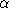## AlphaShapeVertex_2

### Refines

TriangulationVertexBase_2.

### Types

 AlphaShapeVertex_2::FT A type to hold a coordinate type class. The type must provide a copy constructor, assignment, comparison operators, negation, multiplication, division and allow the declaration and initialization with a small integer constant (cf. requirements for number types). An obvious choice would be coordinate type of the point class.

### CreationadvancedAlphaShapeVertex_2 v; default constructor. AlphaShapeVertex_2 v ( Point p); constructor setting the point. AlphaShapeVertex_2 v ( Point p, void* f); constructor setting the point associated to and an incident face.advanced### Access Functions

 std::pair< FT, FT > v.get_range () returns two alpha values12, such as forbetween1 and2, the vertex is attached but singular, and forupper2, the vertex is regular.

### Modifiersadvancedvoid v.set_range ( std::pair< FT, FT > I) sets the alpha values12, such as forbetween1 and2, the vertex is attached but singular, and forupper2, the vertex is regular.advanced